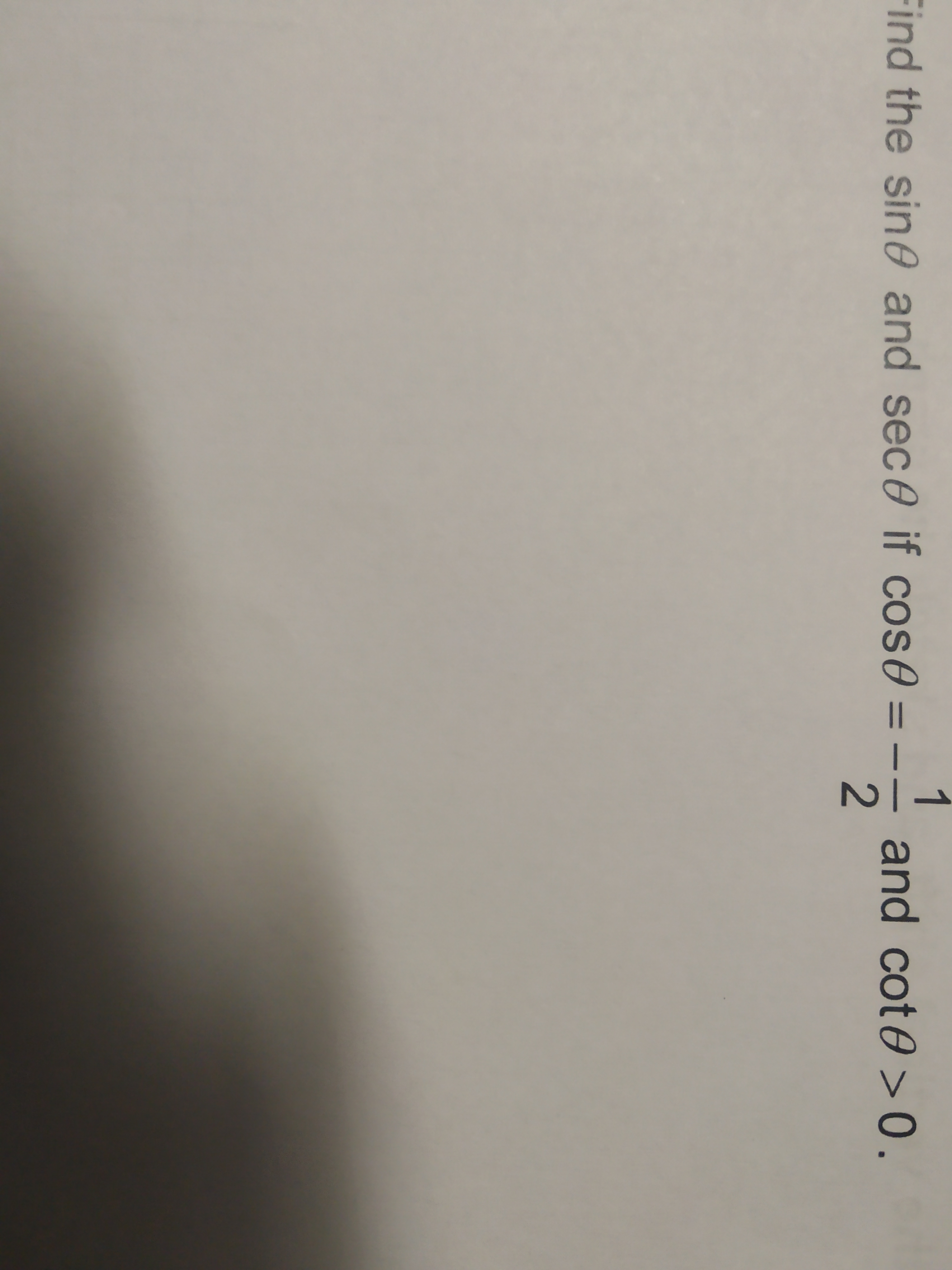Question
43 views

Use identities to find the value of the expressionhelp_outlineImage Transcriptionclose2 ind the sin θ and sec θ if cos6 and cot θ > 0. fullscreen
check_circle

Step 1

Calculation of sec(theta):

we can use formula

sec(theta)=1/cos(theta)

Step 2

Calculation of sin(theta):

We can use trigonometric identities

sin^2(theta) +cos^2(theta)=1

We are given cos value as negative

and cot value is positive

So, theta must li...

### Want to see the full answer?

See Solution

#### Want to see this answer and more?

Solutions are written by subject experts who are available 24/7. Questions are typically answered within 1 hour.*

See Solution
*Response times may vary by subject and question.
Tagged in

### Trigonometry